# Simplevis

#### 2021-06-30

``````library(dplyr)
library(simplevis)
library(palmerpenguins)
library(ggplot2)``````

### Purpose

`simplevis` is a package of `ggplot2` wrapper functions that aims to make visualisation easier with less brainpower required.

### Visualisation family types

`simplevis` supports the following families of visualisation type:

bar

``````plot_data <- storms %>%
group_by(year) %>%
summarise(wind = mean(wind))

gg_bar(plot_data,
x_var = year,
y_var = wind)``````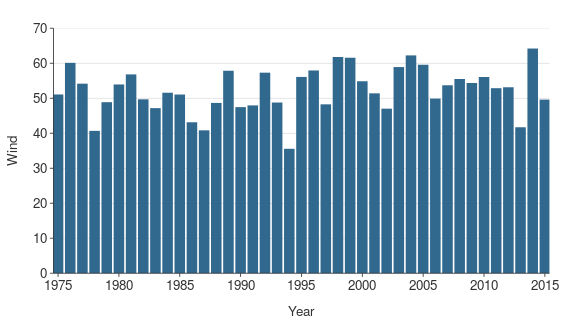point

``````gg_point(iris,
x_var = Sepal.Width,
y_var = Sepal.Length)``````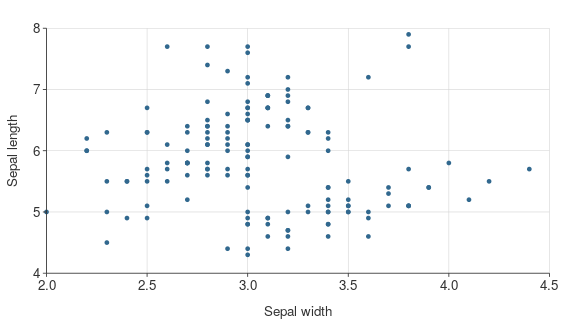line

``````plot_data <- storms %>%
group_by(year) %>%
summarise(wind = mean(wind))

gg_line(plot_data,
x_var = year,
y_var = wind)``````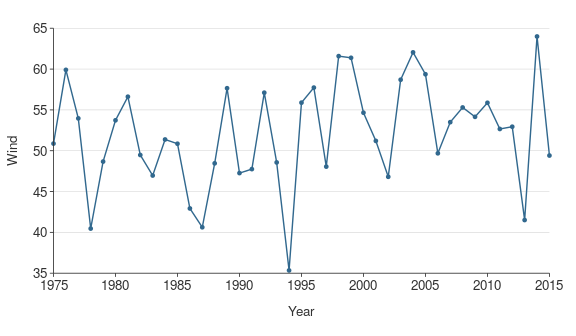hbar (i.e horizontal bar)

``````plot_data <- ggplot2::diamonds %>%
group_by(cut) %>%
summarise(price = mean(price))

gg_hbar(plot_data,
x_var = price,
y_var = cut)``````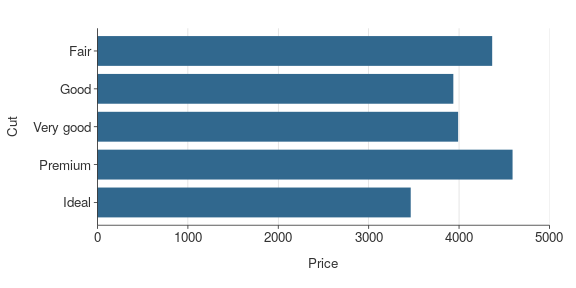density

``````gg_density(penguins,
x_var = body_mass_g)``````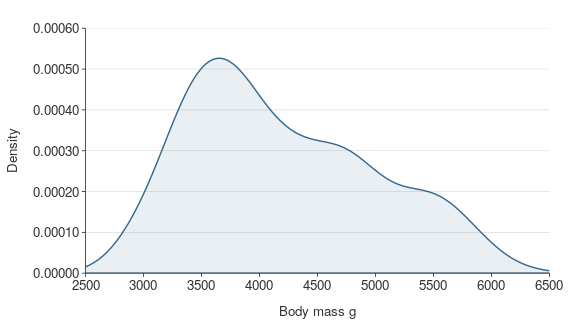boxplot

``````gg_boxplot(storms,
x_var = year,
y_var = wind)``````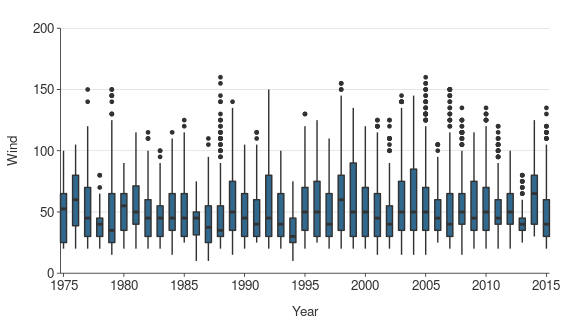sf (short for simple features map)

``````gg_sf(example_sf_point,
borders = nz)``````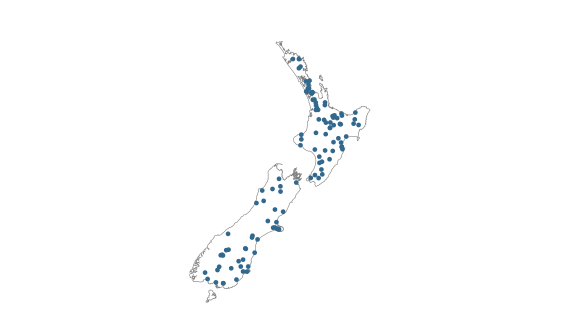### Colouring, facetting, neither or both

Each visualisation family generally has 4 functions.

The function name specifies whether or not a visualisation is to be coloured by a variable (`*_col()`), facetted by a variable (`*_facet()`), or neither (`*()`) or both of these (`*_col_facet()`).

Colouring by a variable means that different values of a selected variable are to have different colours. Facetting means that different values of a selected variable are to have their facet.

A `*()` function such `gg_point()` requires only a dataset, an x variable and a y variable.

``````gg_point(penguins,
x_var = bill_length_mm,
y_var = body_mass_g)``````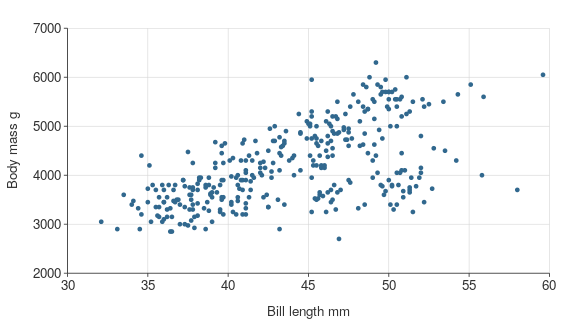A `*_col()` function such `gg_point_col()` requires only a dataset, an x variable, a y variable, and a colour variable.

``````gg_point_col(penguins,
x_var = bill_length_mm,
y_var = body_mass_g,
col_var = sex)``````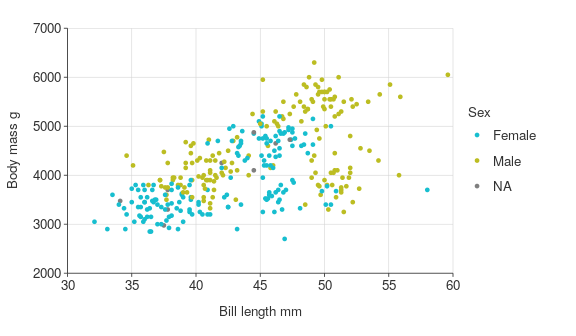A `*_facet()` function such `gg_point_facet()` requires only a dataset, an x variable, a y variable, and a facet variable.

``````gg_point_facet(penguins,
x_var = bill_length_mm,
y_var = body_mass_g,
facet_var = species)``````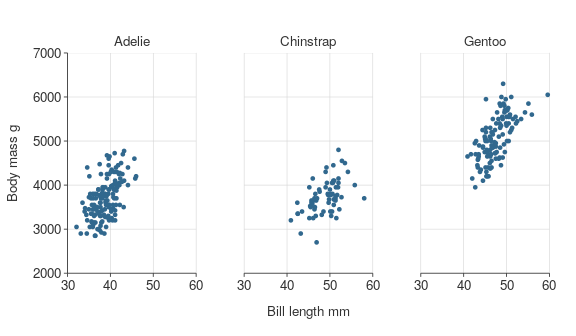A `*_col_facet()` function such `gg_point_col_facet()` requires only a dataset, an x variable, a y variable, a colour variable, and a facet variable.

``````gg_point_col_facet(penguins,
x_var = bill_length_mm,
y_var = body_mass_g,
col_var = sex,
facet_var = species)``````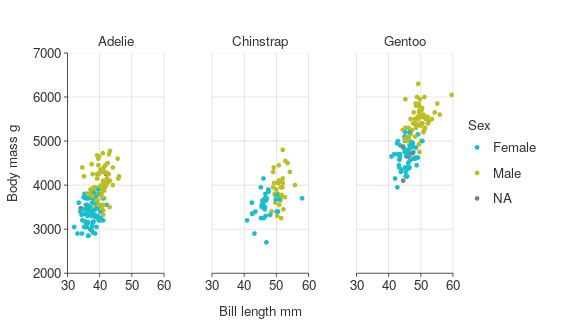Data is generally plotted with a stat of `identity`, which means data is plotted as is.

For boxplot, there is adefault stat of `boxplot`, which means the `y_var` will be transformed to boxplot statistics.

For density, the stat of the `x_var` based on the density prefixed arguments that inform the density calculation.

Generally, an `x_var` and a `y_var` is required. However, `y_var` is not required for `density*()` functions. Neither `x_var` or `y_var` are required for `gg_sf*()` (or `leaflet_sf*()`) functions.

### Titles

Defaults titles are:

• no title, subtitle or caption
• x, y and colour titles are converted to sentence case using the `snakecase::to_sentence_case` function.
``````gg_point_col(penguins,
x_var = bill_length_mm,
y_var = body_mass_g,
col_var = species)``````